Knowing Porogapit, A Long Division for Children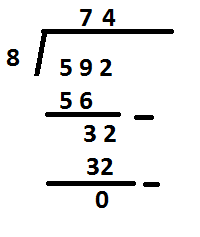The ability to calculate division is useful in everyday life. We can't just look at the calculator. We also have to be able to explain how the distribution is done to our children. This is important so that children feel there is an example from their parents.
There are several ways of sharing that can be done. There is a way of repeated subtraction until it runs out or by stacking.

How to calculate division with whole/integer results with Porogapit.

This time I will present the division in a stacked way.
This long stacking method is known as POROGAPIT Poro means division. Gapit means flanked. You could say Porogapit is a method of division by being flanked by two line blades.

Oh yes, division can be done on the condition that the number divided must be greater than or equal to the divisor. Because a smaller number cannot be divided by a larger number.
Let's look at the following examples of division:
Example!
The result of 592 : 4 is...
We write with the division. Remember, the divisor is on the outside and the divisor is placed inside the porogapit.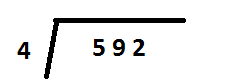Working steps;

• The first step is to look at the digits of the number that is divided. We start from the first digit (5). Because the number 5 is greater than 4 then we can do it directly.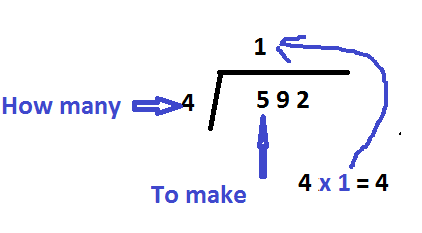• Divide 5 by 4. Or the easiest understanding is 4 times how many times the result is closest to 5 or equal to 5.
5: 4 = 1 remainder 1. We write integers above the square as the first digit of the division result.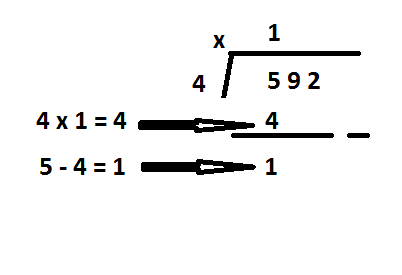• Now multiply 1` by 4 and the result 4 we write under the number 5.
• Next 5 - 4 = 1. The result of subtraction (1) we write below 4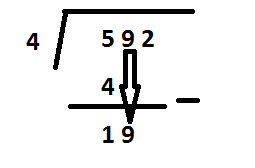• Bring down the copy 9 to the right of 1 so that it becomes 19.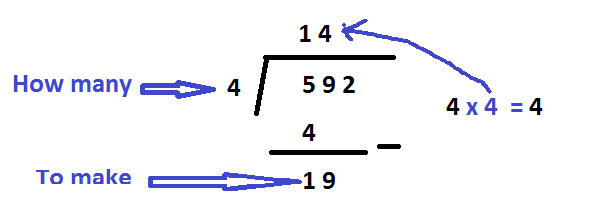• Divide 19 by 4. As in the understanding at the beginning the easiest is 4 times how many times the result is closest to 19 or equal to 19.
19 : 4 = 4 remainder 3. We will write the integer (4) above the angled line as the second digit of the division result.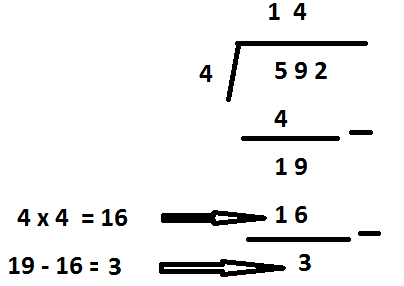• Bring down a copy number 2 to right side 3 so become 32.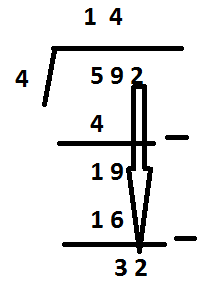• Divide 32 by 4. As understood at the beginning the easiest is 4 times how many times the result is closest to 32 or equal to 32.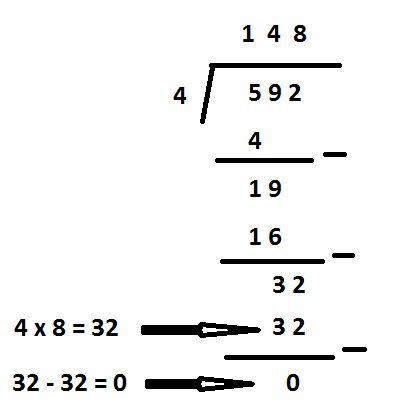It turns out that 4 x 8 = 32.
32 : 4 = 8 without remainder. Then we write the number 8 above the gaping line as the third digit of the division result.
Thus we know the result of the calculation of 592: 4 is 148

Example 2

The result of 592 :8 is...
We can write the division with the following half-closets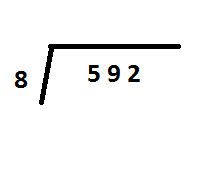Working steps;

• The first step is to look at the digits of the number that is divided. We start from the first digit (5). Because the number 5 is smaller than 8, we use two digits so that the number we divide the first time is 59.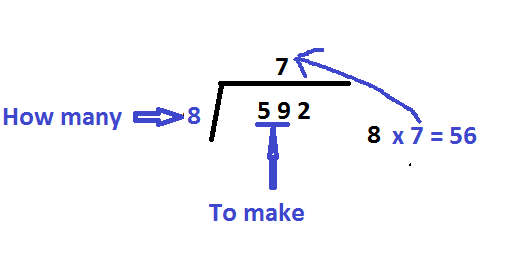• Divide 59 by 4. Or the easiest understanding is 4 times how many times the result is closest to 59 or equal to 59.
59 : 8 = 7 remainder 3. We write integers above the angled line as the first digit of the result of the division.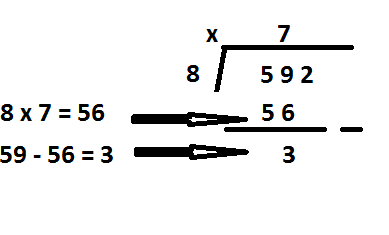• Bring down number 2 to right side 3 so become 32.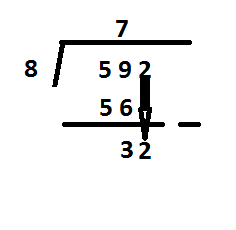• Divide 32 by 8. As explained earlier 8 times how many times the result is closest to 32 or equal to 32.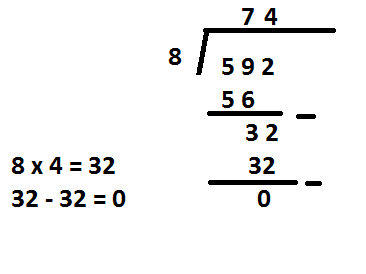It turns out that 8 x 4 = 32.
32 : 8 = 4 without remainder. So we write the number 4 above the gaping line as the second digit of the division result.
Thus we know the result of the calculation of 592: 8 is 74

Thus my explanation of the downward tiered division known as Porogapit may be useful for all of us.
Best regard from Indonesia
@rokhani

0
0
0.000